###### Indexed In
• Genamics JournalSeek
• RefSeek
• Hamdard University
• EBSCO A-Z
• OCLC- WorldCat
• Publons
• Euro Pub
###### Journal Flyer###### Open Access Journals

Research Article - (2016) Volume 5, Issue 1

# Robust PID Tuning of Autonomous Underwater Vehicle Using Harmonic Search Algorithm Based on Model Order Reduction

Ankush Rathore* and Mahendra Kumar
Department of Electronics University College of Engineering, RTU Kota, Rajasthan, India, E-mail: [email protected]
*Corresponding Author: Ankush Rathore, Department of Electronics University College of Engineering, RTU Kota, Rajasthan, India, Tel: 9180003308800 Email:

## Abstract

The objective of this paper is to present Genetic Algorithm (GA) and Harmonic Search Algorithm (HSA) based on PID tuning and Model Order Reduction with Balance Truncation (MORBT). This method is used for constructing a second order balanced truncation model order reduction for symmetric linear time invariant third order control systems. These methods are closely related to the proper and improper controllability and observability Gramians and Hankel singular values of descriptor systems. The HS (Harmonic Search) algorithm mimics behaviours of music players in an improvisation process, in order to find a better state of harmony which can be translated into a solution vector in the optimization process. PID (proportional-integral-derivative) controller provides sufficient stability margins and good time responses. It is now possible to design an optimal PID controller with properties of a GA and HSA. In control strategies, like PID controller are successfully designed to control the autonomous underwater vehicle. The elementary focus is to simulate the controller response.

Keywords: Autonomous underwater vehicle, Genetic algorithm,Harmonic search algorithm, Model order reduction by balance truncation,Simulation,System identification

#### Introduction

AUV refers to an autonomous underwater vehicle equipped with suitable sensors and actuators which enable to navigate through unknown environments while performing certain user specified tasks. AUV’s have 3 degrees of freedom and the subsystem is coupled with strong interaction . It executes the assigned mission without any external human intervention. Some applications are surveillance, navigation and monitor. Horner and Goldberg  applied a GA model to bridge-music composition in the minimalist style. It turns out that Harmony Search is a special case of Evolution Strategies. The HS algorithm initializes the Harmony Memory (HM) with randomly generated solutions. The number of solutions stored in the HM is defined by the Harmony Memory Size (HMS) (Table 1) (Figure 1). The parameters that are used in the generation process of a new solution are called Harmony Memory considering Rate (HMCR) and Pitch Adjusting Rate (PAR). This study, another evolutionary in algorithm (harmony search or HS), inspired by music improvisation, is applied to music composition. The proposed HS algorithm was created by analogy to the music improvisation process. It is known that AUV systems also include uncertainties.Thus, robustness analysis of the system becomes very important and must be considered. In this paper in order to achieve a more realistic design, the robust stability region of the system is identified by using the GA (Genetic algorithm). GA is a heuristic that mimics the process of natural selection  which determines the performance of the genetic algorithm performs is the diversity of the population .One of the first proponents John Holland in 1975 . A collection of a number of individuals is called a population . The basic goal of GA is to optimize functions called fitness functions and evaluate by this function . However, the melody developed by GA could not be evaluated because there was no appropriate fitness function. The HS was superior to the GA in most cases because it overcame the drawback of the building block theory of GA . This paper is organized as follows. Section II, modelling AUV system is presented. Then in Section III design and tuning of PID controller using HSA and GA is described. Section IV model order reduction by balance truncation is described. Section V, shows the simulation results. Finally, Section VI gives conclusions.

#### Generalized Mathematical Modelling of AUV

A further goal is the consideration of coordinated control designs for multiple AUV applications. In that case, the particular limitations in underwater sensing and communication of the AUV team should be taken into consideration. Modelling of the proposed controller on a nonlinear six degrees of freedom (DOF), wherein only four DOF (x, y, z, p) are actuated and the rest of them are considered intrinsically stable. This paper discuses tuning of PID controller using genetic algorithm (GA).

Kinetic equation of motion of AUV

A generic 6 degree of freedom suitable for AUV control application with respect two reference frames, i.e., earth-fixed frame and body-fixed frame .The earth coordinate system is defined by three orthogonal axes originating at an arbitrary point. North corresponds to x-axis, East corresponds to y-axis and increasing depth corresponds to z-axis. The velocity parameters of the AUV are determined from the body-fixed frame and using a transfer- motion matrix, the velocity in the earthfixed frame is determined.(1)(2)

The simplified equation of motion in pure steering plane is written by assuming the origin of body fixed frame to coincide with centre of gravity as: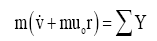(3)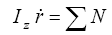(4)

Surge speed as constant (u0 =0.75 m/s). For small roll and pitch angle have:(5)

The above equations are expressed in a matrix form as:(6)

Using value of the dimensions, hydrodynamic coefficient and vehicle parameter of NPS AUV II for u0 =0.75 m/s  have:(7)

Where,The transfer function between yaw (ψ ) and rudder deflection (δr ) is obtained as:(8)

#### PID Tuning using Harmonic Search Algorithm and GA

Harmonic search algorithm (HSA)

Harmony search (HS) is a relatively new population-based music inspired optimization algorithm that imitates the music improvisation process and has been applied to various optimization problems includes sudoku, puzzle, logistic, robotics, medical. Music improvisation seeks to produce an ideal state as determined by aesthetic estimation. Similarly, algorithmic optimization seeks to produce an ideal state as determined by objective function evaluation.Recently, HS algorithm was also applied to astronomical data analysis, which was published in Nature .The harmony memory (HM) is a matrix of solutions with a size of HMS (Harmony Search size).In this step, the solutions are randomly constructed and rearranged in a reversed order to HM, based on their objective function values:(9)

Fitness Value=min (J)       (Table 2) (Figure 2)• HMCR (Harmony Memory Considering Rate) –The rate choosing a value from HM and varies from 0.7 to 0.99.

• PAR (Pitch Adjust Rate) –The rate choosing a neighbourhood value and varies from 0.1 to 0.5.

• HMS (Harmony Memory Size) –Size of the HM and varies from 1 to 100.

Pseudo code for the HS algorithm 

Begin

Define Fitness unction f (a) a= (a1,a2,……….aN)T

Define (HMCR), (PAR)

Define maximum number of iteration (NI)

HM←Generate Initial Population ()

Minimum=Minimum Visible Value

Maximum=Maximum Visible Value

While (iter ≤ N1) do

While (ai ≤ number of variables) do

If (rand ∈(0, 1) ≤ HMCR) Then

Choose a value from HM for i

If (rand ∈(0, 1) ≤ PAR) Then

Adjust the value of i by;

ainew=aiold+r and ∈(0, 1) xbw

End if

Else

Choose a random variable

ai=min+rand ∈(0, 1) × (max-min)

End if

End while

If (Fit Fun (New Harmony solution)) ≤ worst (Fit Fun (HM))) Then

Accept the New Harmony and replace the worst in HM with it

End if

End while

Best=fine the current best solution

End

Genetic algorithm (GA)

In respect MATLAB/SIMULINK is a best tool used for it. PID controller is successfully implemented in control systems that provide sufficient stability margins and good time responses. Here PID controller is designed to study the behaviour of AUV. This paper discuses tuning of PID controller using genetic algorithm (GA).The current population reproduces new individuals that are called the new generation. The new individuals of the new generation are supposed to have better performance than the individuals of the previous generation .

Fitness function: Fitness approximation may be appropriate, especially in the following cases:

• Fitness computation time of a single solution is extremely high.

• Precise model for fitness computation is missing.

• The fitness function is uncertainty.

The fitness function is used to provide a measure of how individuals have performed in the problem domain .The most crucial step in applying GA is to use the fitness function that is used to evaluate fitness of each chromosome. The fitness not only indicates how good the solution is but also corresponds to how close the chromosome it to the optimal one. Here in this paper integral of square of error is used as a fitness function. It is given as:(10)

Fitness value: The PID controller is designed to study the behaviour of AUV and more rigorously in the term of error criteria to minimize the value of performance indices and because of the smaller the value of performance indice of the corresponding chromosome the fitter the chromosome will be vice-versa the fitness of chromosome as:

Fitness Value=min (J) (Table 3) (Figure 3)                               (11)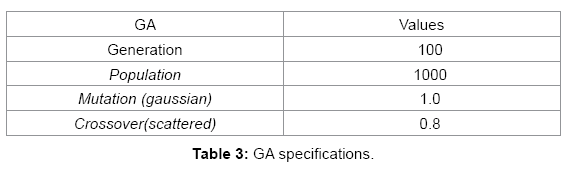#### Modal Order Reduction by Balanced truncation

Plant-order truncation

Balance truncation is a well-established tool for deriving reduced model that have an input-output behaviour similar to the original model [14,15]. The general idea of balanced truncation is to restrict the system onto the subspace of easily controllable and observable states which can be determined by the computing the Hankel singular values associated with the system. The main objective of balance method is constructing a reduced system by preserving the second-order Structure of the original system and compares it with another existing technique. Second order balance reduction (SOBR) is superior to the pioneering work in the control community because second-order systems can be balanced via congruency transformation without any accuracy loss. In addition, compared with the first-order balanced truncation approaches, SOBR is a better choice for second order reduction.

For first-order system(12)(13)

Balanced truncation method is to transform the system to another coordinate system such that both P and Q are equal and diagonal:(14)

Then truncates the model by keeping the states corresponding to k largest eigenvalues of the product PQ, that is, k largest Hankel singular values of the system. The main problem is to find balancing projection matrices. Given a system in a standard second-order form:(15)(16)

Where, y(t)∈ Rp , y(t)∈ Rp , q(t)∈ Rnsecond-order gramians in  are defined based on a first-order realization (1) with 2n-dimensional state xT= [qT q?T] ,

Where,The first-order realization  has the same input-output behavior as the second-order system . Due to limitations on time and computer storage, it is often difficult or impossible to directly simulate or control these large-scale systems. Therefore, it is often desirable to reduce the system to a smaller-order model:(17)(18)

Where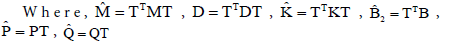Which is not really balanced because TT instead of T-1 is used to preserve the symmetry and thus stability of the original system? Such that

• The reduced system preserves system properties such as stability and passivity.

• The procedure is computationally efficient.

Hankel singular values

Similar to state space system  the controllability and observability Gramians can be used to define Hankel singular values for the descriptor system. Those are of great importance in model reduction via balanced truncation.

SISO third–order system

We consider an SISO third-order system  described by the trasnfer function,(19)

Then, reduced order plant is obtained as(20)

Thus, SBPOR (Second-order Balanced truncation for Passive Order Reduction), for the model order reduction of AUV system. By fully utilizing the symmetric positive definiteness of the system matrices, new second-order gramians are defined based on a symmetric firstorder realization in descriptor form so that the second-order system can be really balanced via congruency transformation without any accuracy loss. Experimental results show that SBPOR is more accurate than existing second-order balanced truncation technique.

#### Simulation and Results

The Simulation result for AUV control for the PID controller using GA and PID controller using HSA is shown in Figure 4 and 5 respectively. Figure 6 shows unit step response by resultant controller along with the results by . The final values of PID parameters used for AUV control are given in Table 4. The transient performance of PID using GA and HSA is given in Table 5. The cost comparison between the GA and HSA is given in Table 6. The evolution of PID controller by GA Balance Truncation and HSA Balance Truncation is shown in Figure 7 and 8 respectively. This paper addressed the path following control problem of an Autonomous Underwater Vehicle (AUV). Simulation shows that the control is able to provide reasonable depth and heading station keeping control. From the above simulation result it can be see that the AUV is tuning its desired path (Figure 4).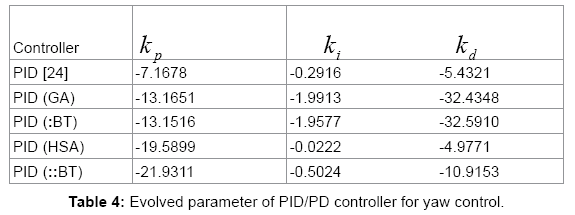#### Conclusion

In this paper, a preserving model order reduction method for second-order systems has been discussed. The reduced model is then obtained by keeping that part of the balanced system which automatically gives a reduced model of second-order type. PID controller parameters that guarantee stability of the system are computed by harmonic search algorithm GA(Table 4-6).

Numerically speaking our method performs better than the method of , but for symmetric model, both methods work well. Now looking towards simulation results, designed the PID controller, which yields good results to stable AUV system. It is seen that the response of yaw for PID using HSA is better than PID using GA. Important properties of this method illustrates the regularity and stability is preserved in the reduced order system but there is an priori bound on the approximation error. The effectiveness of the proposed model reduction algorithms has been demonstrated by numerical experiments (Figure 5-8).

#### References

1. Goheen KR, Jefferys E (1990) “The application of alternative modeling technique to ROV dynamics” in proceedings of IEEE international conference on Robotics and Automation 2:1302-1309
2. Johnson CG, Cardalda JJR (2002) Genetic Algorithms in Visual Art and Music. Leonardo 35:175-184
3. Goldberg DE (1989) Genetic algorithms in search, optimization, and machine learning, Addison Wesley Longman, Inc., ISBN 0-201-15767-5
4. Rajib Kumar Bhattacharyya, Introduction to Genetic Algorithm, Department of Civil Engineering IIT Guwahati
5. Holland J (1975) Adaptation in natural and artificial systems, University of Michigan Press, Ann Arbor
6. David E Goldberg (1989) “Genetic algorithms in search, optimization and machine learning,” Addison Wesley
7. Elisaveta G Shopova, Natasha G, VaklievaBancheva (2005)“BASIC-A genetic algorithm for engineering problems solution” Elsevier journal 1:17
8. Geem ZW (2006) Improved Harmony Search from Ensemble of Music Players. Lecture Notes in Artificial Intelligence 4251:86-93
9. Fossen T (1994) Guidance and Control of Ocean Vehicles. New York: Wiley 2:6–55
10. Kadam SD, Tiwari KN (2013) “A simplified approach to tune PD controller for depth control of an autonomous underwater vehicle” in proceedings of National conference on Communication, Computing and Networking Technologies 209-212
11. Tasgetiren J (2010). A local-best harmony search algorithm with dynamic subpopulations. Engineering Optimization 42:101-117
12. Jin-Sung Kim, Jin-Hwan Kim, Ji-Mo Park, Sung-Man Park, Won-Yong Choe et al. “Auto tuning PID controller based on improved Genetic Algorithm for reverse osmosis plant,” World academy of Science, Engineering and Technology 384-389
13. Liu fan, ErMeng Joo (2009) “Design for auto-tuning PID controller based on Genetic Algorithms” IEEE conference on Industrial Electronics and application 1924-1928.
14. Zhou K, Doyle JC, Glover K (1998) Robust and Optimal Control. Prentice Hall, New Jersey.
15. Antoulas AC (2005) Approximation of Large-Scaled Dynamical Systems. SIAM
16. Meyer DG, Srinivasan S (1996) “Balancing and model reduction for second-order form linear systems,” IEEE Trans. Automat Contr 41: 1632–1644
17. Pillage LT, Rohrer RA (1990) “Asymptotic waveform evaluation for timing analysis,” IEEE Trans. On Computer-Aided Design of Integrated Circuits and Systems 9: 352-366
18. Abhay A, Pakhare, Kunal N Tiwari, Rajan H Chile (2013) “PID/PD tuning for Steering Control of Autonomous Underwater Vehicle: Genetic Algorithm Approach”, Proceedings of National conference on Instrumentation, Control and Signal Processing (ICSP-2013).
Citation: Rathore A, Kumar M (2015) Robust PID Tuning of Autonomous Underwater Vehicle Using Harmonic Search Algorithm Based on Model Order Reduction. Int J Swarm Intel Evol Comput 4:127.

Copyright: © 2015 Rathore A, et al. This is an open-access article distributed under the terms of the Creative Commons Attribution License, which permits unrestricted use, distribution, and reproduction in any medium, provided the original author and source are credited.